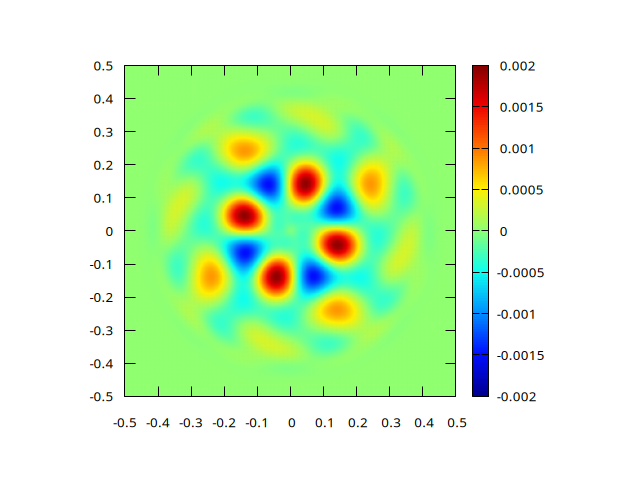# Pure rotation of a smooth tracer field

set output 'error.png'
set pm3d
set pm3d map interpolate 1,1
set palette defined ( 0 0 0 0.5647, 0.125 0 0.05882 1, 0.25 0 0.5647 1,\
0.375 0.05882 1 0.9333, 0.5 0.5647 1 0.4392, 0.625 1 0.9333 0, \
0.75 1 0.4392 0, 0.875 0.9333 0 0, 1 0.498 0 0 )
set size ratio -1
splot 'out' t ""Error field after one rotation for N=256 (script)

reset
ftitle(a,b) = sprintf("%.0f/x^{%4.2f}", exp(a), -b)
f(x)=a+b*x
fit f(x) 'log' u (log($1)):(log($4)) via a,b
f2(x)=a2+b2*x
fit f2(x) 'log' u (log($1)):(log($2)) via a2,b2
set xlabel 'Maximum resolution'
set ylabel 'Maximum error'
set logscale
set cbrange [1:2]
set xrange [32:512]
set xtics 32,2,512
set grid ytics
plot 'log' u 1:4 t 'max', 'log' u 1:2 t 'norm1', \
exp(f(log(x))) t ftitle(a,b), exp(f2(log(x))) t ftitle(a2,b2)Error as a function of resolution (script)

We use the advection solver with a single tracer f.

#include "grid/cartesian.h"

scalar f[];
scalar * tracers = {f};

We impose no normal flux on the box boundaries.

u.n[left]   = 0.;
u.n[right]  = 0.;
u.n[top]    = 0.;
u.n[bottom] = 0.;

int main()
{
// coordinates of lower-left corner
origin (-0.5, -0.5);
// maximum timestep
DT = .1;
// CFL number
CFL = 0.8;

The spatial resolution varies from 64 to 256 points per box size to study spatial convergence.

  for (N = 64; N <= 256; N *= 2)
run ();
}

The initial tracer field is a somewhat complicated but compact and smooth function.

double bump (double x, double y)
{
double r2 = x*x + y*y;
double coeff = 20. + 20000.*r2*r2*r2*r2;
return (1. + cos(20.*x)*cos(20.*y))*exp(-coeff*r2)/2.;
}

event init (i = 0)
{
foreach()
f[] = bump(x,y);
}

The advection velocity field is a simple solid rotation.

event velocity (i++) {
trash ({u});
foreach_face(x) u.x[] = -8.*y;
foreach_face(y) u.y[] =  8.*x;
}

We log statistics on mass conservation and errors after one full rotation.

#define end 0.785398
event logfile (t = {0,end}) {
stats s = statsf (f);
fprintf (stderr, "# %f %.12f %g %g\n", t, s.sum, s.min, s.max);
}

event field (t = end) {
scalar e[];
foreach()
e[] = f[] - bump(x,y);
norm n = normf (e);
fprintf (stderr, "%d %g %g %g\n", N, n.avg, n.rms, n.max);

if (N == 256)
output_field ({e}, stdout, N);
}